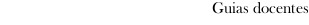Guia docente 2020_21Facultad de Ciencias del Mar
 Grao en Ciencias do MarSubjectsMathematics: Mathematics IIContents
 Topic Sub-topic Integral of line. Fields *conservativos Regular curves. Integral along a curve. Work realized by a field. Fields *conservativos. *Rotacional. Divergence Double integration. Surfaces. Integration in *rectángulos. Integration in general areas. Change of variable. Polar coordinates. *Teorema Of *Green. Parametric and regular surfaces. Orientation of a surface. Integral of surface. Integration @triple. Integral of flow. *Teoremas Of Stokes. Integration @triple. Spherical coordinates and *cilíndricas. *Teorema Of *Gauss. Differential equations of first order Solution of a differential equation. Equations in separate variables. Exact equations. Linear equations. Linear differential equations of upper order Linear equations of order *n. Solutions. Linear equations with @constante coefficients. General solution of the equation *homoxénea. Particular solution of the complete equation. *Temario Of laboratory Resolution of exercises of integration and differential equations by means of programs of calculation.
 Universidade de Vigo            | Rectorado | Campus Universitario | C.P. 36.310 Vigo (Pontevedra) | España | Tlf: +34 986 812 000